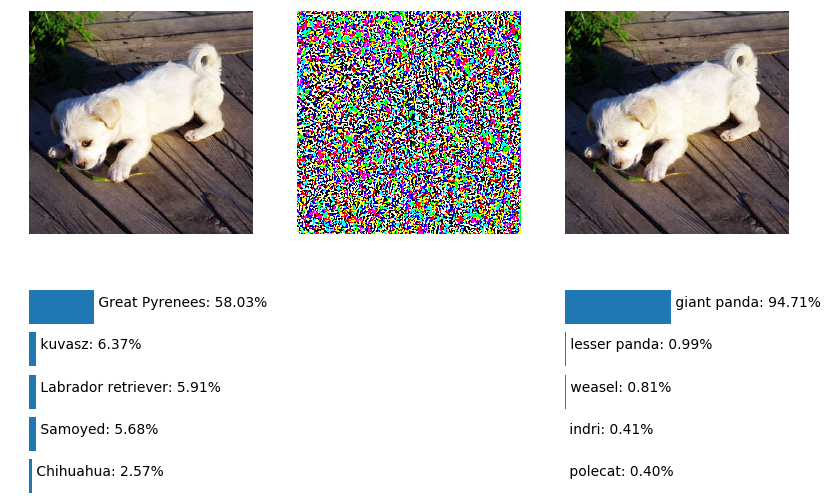## 用Caffe生成对抗样本

FGS法因为非常简单，用任何框架都很容易实现（Ian Goodfellow有个作为完整工具包的官方实现，基于TensorFlow：openai/cleverhans），这里给出Caffe的Python接口实现的例子。

force_backward: true


# model to attack
model_definition = '/path/to/deploy.prototxt'
model_weights = '/path/to/squeezenet_v1.0.caffemodel'
channel_means = numpy.array([104., 117., 123.])

# initialize net
net = caffe.Net(model_definition, model_weights, caffe.TEST)
n_channels, height, width = net.blobs['data'].shape[-3:]
net.blobs['data'].reshape(1, n_channels, height, width)

# initialize transformer
transformer = caffe.io.Transformer({'data': net.blobs['data'].data.shape})
transformer.set_transpose('data', (2, 0, 1))
transformer.set_mean('data', channel_means)
transformer.set_raw_scale('data', 255)
transformer.set_channel_swap('data', (2, 1, 0))


# Load image & forward
transformed_img = transformer.preprocess('data', img)
net.blobs['data'].data = transformed_img
net.forward()

# Get predicted label index
pred = numpy.argmax(net.blobs['prob'].data.flatten())

# Set gradient direction to reduce the current prediction
net.blobs['prob'].diff[pred] = -1.

# Generate attack image with fast gradient sign method
diffs = net.backward()
diff_sign_mat = numpy.sign(diffs['data'])


net.blobs[prob_blob].diff[label_index] = 1.


# clip exceeded values
attack_hwc = transformer.deprocess(data_blob, transformed_img + adversarial_noise)
attack_hwc[attack_hwc > 1] = 1.
attack_hwc[attack_hwc < 0] = 0.
attack_img = transformer.preprocess(data_blob, attack_hwc)


attack_img就是和Caffe的blob形状一致的对抗样本了，attack_hwc是维度按照图片高度，图片宽度，图片通道顺序的格式，可以用matplotlib直接可视化。

## 可视化和简单分析

def make_n_test_adversarial_example(
img, net, transformer, epsilon,
data_blob='data', prob_blob='prob',
label_index=None, top_k=5):

transformed_img = transformer.preprocess(data_blob, img)
net.blobs[data_blob].data = transformed_img
net.forward()
probs = [x for x in enumerate(net.blobs[prob_blob].data.flatten())]
num_classes = len(probs)
sorted_probs = sorted(probs, key=itemgetter(1), reverse=True)
top_preds = sorted_probs[:top_k]
pred = sorted_probs

# if label_index is set,
# generate a adversarial example toward the label,
# else
# reduce the probability of predicted label
net.blobs[prob_blob].diff[...] = 0
if type(label_index) is int and 0 <= label_index < num_classes:
net.blobs[prob_blob].diff[label_index] = 1.
else:
net.blobs[prob_blob].diff[pred] = -1.

# generate attack image with fast gradient sign method
diffs = net.backward()
diff_sign_mat = numpy.sign(diffs[data_blob])

# clip exceeded values
attack_hwc = transformer.deprocess(data_blob, transformed_img + adversarial_noise)
attack_hwc[attack_hwc > 1] = 1.
attack_hwc[attack_hwc < 0] = 0.
attack_img = transformer.preprocess(data_blob, attack_hwc)

net.blobs[data_blob].data = attack_img
net.forward()
probs = [x for x in enumerate(net.blobs[prob_blob].data.flatten())]
sorted_probs = sorted(probs, key=itemgetter(1), reverse=True)
top_attacked_preds = sorted_probs[:top_k]

return attack_hwc, top_preds, top_attacked_preds


def visualize_attack(title, original_img, attack_img, original_preds, attacked_preds, labels):
pred = original_preds
attacked_pred = attacked_preds
k = len(original_preds)
fig_name = '{}: {} to {}'.format(title, labels[pred], labels[attacked_pred])

pyplot.figure(fig_name)
for img, plt0, plt1, preds in [
(original_img, 231, 234, original_preds),
(attack_img, 233, 236, attacked_preds)
]:
pyplot.subplot(plt0)
pyplot.axis('off')
pyplot.imshow(img)
ax = pyplot.subplot(plt1)
pyplot.axis('off')
ax.set_xlim([0, 2])
bars = ax.barh(range(k-1, -1, -1), [x for x in preds])
for i, bar in enumerate(bars):
x_loc = bar.get_x() + bar.get_width()
y_loc = k - i - 1
label = labels[preds[i]]
ax.text(x_loc, y_loc, '{}: {:.2f}%'.format(label, preds[i]*100))

pyplot.subplot(232)
pyplot.axis('off')
noise = attack_img - original_img
pyplot.imshow(255 * noise)


attack_img, original_preds, attacked_preds = \
visualize_attack('example0', img, attack_img, original_preds, attacked_preds, labels)


attack_img, original_preds, attacked_preds = \
visualize_attack('example1', img, attack_img, original_preds, attacked_preds, labels)


## 利用迭代更好地生成对抗样本

attack_img, original_preds, attacked_preds = \